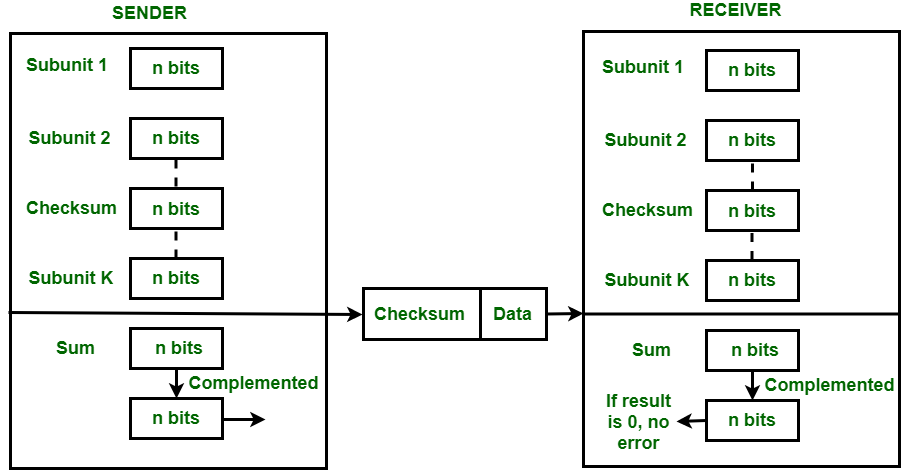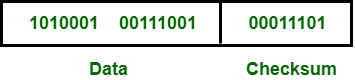# Error Detection Code – Checksum

• Difficulty Level : Easy
• Last Updated : 16 Jul, 2021

Prerequisite – Error Detection in Computer Networks

Checksum is the error detection method used by upper layer protocols and is considered to be more reliable than LRC, VRC and CRC. This method makes the use of Checksum Generator on Sender side and Checksum Checker on Receiver side.

At the Sender side, the data is divided into equal subunits of n bit length by the checksum generator. This bit is generally of 16-bit length. These subunits are then added together using one’s complement method. This sum is of n bits. The resultant bit is then complemented. This complemented sum which is called checksum is appended to the end of original data unit and is then transmitted to Receiver.The Receiver after receiving data + checksum passes it to checksum checker. Checksum checker divides this data unit into various subunits of equal length and adds all these subunits. These subunits also contain checksum as one of the subunits. The resultant bit is then complemented. If the complemented result is zero, it means the data is error-free. If the result is non-zero it means the data contains an error and Receiver rejects it.

Example –
If the data unit to be transmitted is 10101001 00111001, the following procedure is used at Sender site and Receiver site.

Sender Site :

```10101001        subunit 1
00111001        subunit 2
11100010        sum (using 1s complement)
00011101        checksum (complement of sum)```

Data transmitted to Receiver is –```10101001        subunit 1
00111001        subunit 2
00011101        checksum
11111111        sum
00000000        sum's complement

Result is zero, it means no error.```

The checksum detects all the errors involving an odd number of bits as well as the error involving an even number of bits.

The main problem is that the error goes undetected if one or more bits of a subunit is damaged and the corresponding bit or bits of a subunit are damaged and the corresponding bit or bits of opposite value in second subunit are also damaged. This is because the sum of those columns remains unchanged.

Example –
If the data transmitted along with checksum is 10101001 00111001 00011101. But the data received at destination is 00101001 10111001 00011101.

```00101001              1st bit of subunit 1 is damaged
10111001              1st bit of subunit 2 is damaged
00011101              checksum
11111111              sum
00000000              Ok 1's complement```

Although data is corrupted, the error is undetected.

My Personal Notes arrow_drop_up